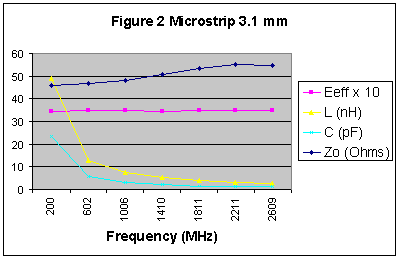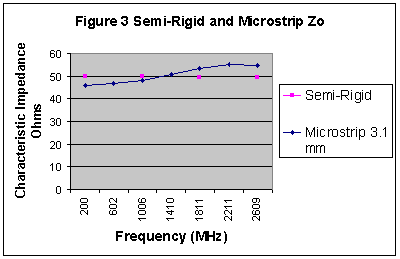# Measuring Characteristic Impedance and Effective Dielectric Constant of PCB tracks using a Vector Network Analyser page 3

Removing the Ripples

The results at +900 and -900 are averaged by using Formula 1 for complex numbers, and simply adding together and dividing the sum by two for the others . This removes the ripples and the result is more representative of the measurement at 00. The effect of this is shown in Figure 2. Note that not only have the ripples been removed, but the first inductive measurement has dropped considerably also.Proof

There is a rise in impedance with frequency that could be a measurement error. In order to first eliminate this, a short piece (14 cm) of semi-rigid cable was measured by the same method and the result is compared with the microstrip in Figure 3.It can be seen that the Semi-Rigid gives virtually a straight line at 50 Ohms, as would be expected of a cable of this quality. This proves that the measurement method does not introduce a slope and the rise in characteristic impedance with frequency for the measured microstrip is real. It is interesting to note that the microstrip is 50 Ohm at about 1400 MHz, and this is the sort of frequency that would correspond to the typical measurement rise time of a TDR. It would appear therefore, that the formula by Brian C Wadell which was used to calculate the Zo, is accurate only at the frequency typically measured by a TDR. This would also be true for the other two formulas used for our microstrip calculators.

Comparison of Results With Return Loss Measurements

Many return loss measurements of microstrip transmission line have been made in a FLEXI-BOX, which has a track length of 5.5 cm. This is not long enough to make characteristic impedance measurements, but is suitable data for comparison. The characteristic impedance of the 3.1 mm sample displayed in Figure 2 was used to approximate the expected return loss of the microstrip with the two mismatches that would be present with two connectors as used in the return loss measurement. This is compared with a typical return loss measurement made with a 3.0 mm microstrip fitted in a FLEXI-BOX in Figure 4.There is good correlation between these curves which can be understood with the aid of the following analyses.

Firstly, consider the portion of curve below 600 MHz. The measurement in the FLEXI-BOX has a much better return loss than the calculated values. This is because the track has not yet started acting as a transmission line. The 3.0 mm wide track in the box is basically just a very small inductor between the input and output measurement ports at 0.3 MHz. At this frequency, a two inch nail would give similar results! As the frequency is increased, the impedance of the track increases until it behaves like a transmission line at 600 MHz.

The curve down from 600 MHz to 1400 MHz is indicative of the Zo rising from a calculated 47.4 Ohms at 600 MHz to the measurement system impedance of 50 Ohms. The return loss levels do not match exactly, but the minimum occurs at the same frequency which indicates both tracks are 50 Ohms at the same frequency.

From 1400 MHz upwards, the Zo increases from 50 Ohms until it reaches a calculated value of 54.19 Ohms at 2600 MHz. The shape of the curves matching quite well.

It is reasonable to assume from these observations that the rise of impedance over frequency and it's passing through the system impedance of 50 Ohms at approximately 1400 MHz are all real. It is a simple comparison using basic principles and needs no complicated calculations or advanced simulations.

Conclusions

1) The method is valid and gives results that compare favourably with previous return loss measurements.

2) The data obtained over a frequency sweep with a VNA is more useful than that which can be obtained with a TDR measurement, because it is possible to select a more accurate impedance at the required working frequency.

3) The method is suitable for coaxial cables.

The Characteristic Impedance Calculator for the Measurement of PCB Tracks and Coaxial Cables Using a Vector Network Analyser is accurate, but it is a little tedious to enter the large amount of data required for a full frequency sweep and it is necessary to check data entry carefully before calculation. If a large number of measurements are made and the results are required in a chart, it is preferable to use a spreadsheet.

William Jeffrey Highton 16/1/2005

1  2  3  >  Page

Technical Article Index